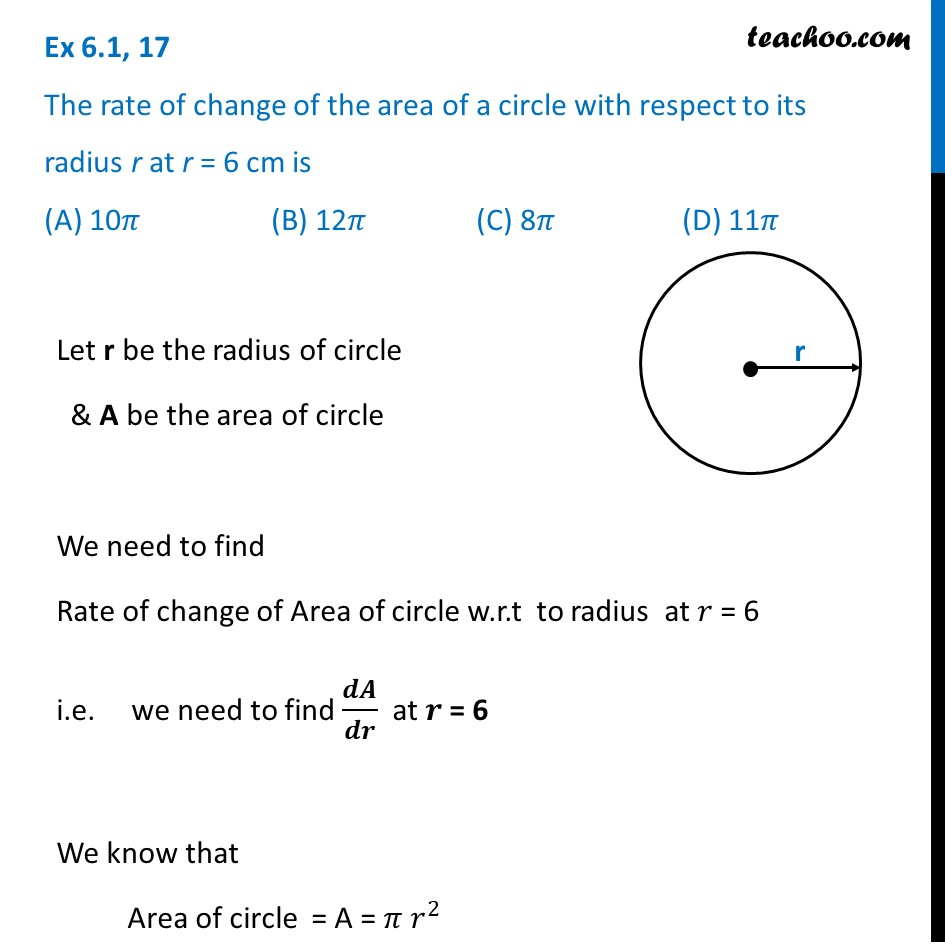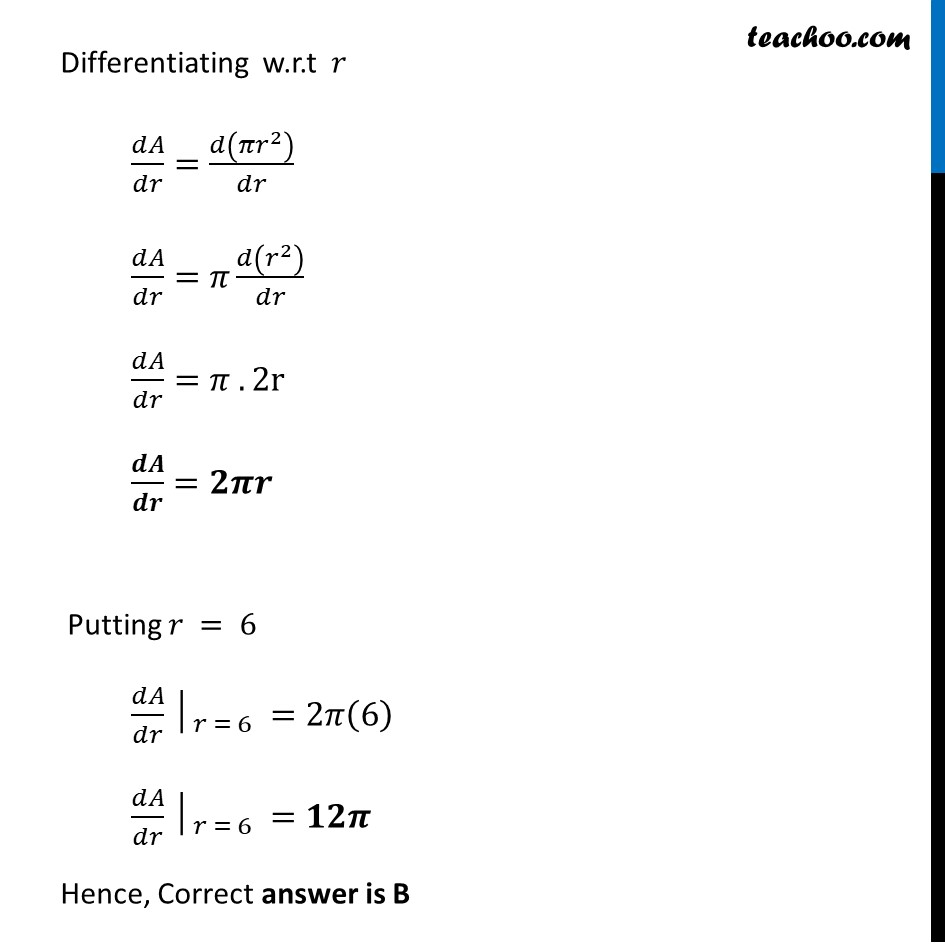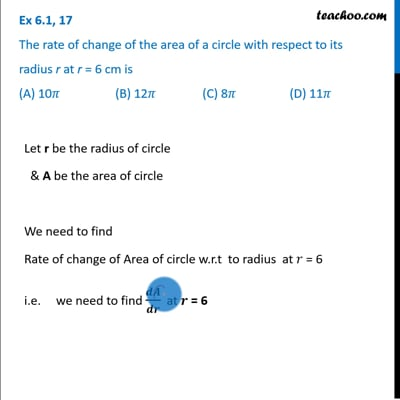Ex 6.1

Chapter 6 Class 12 Application of Derivatives
Serial order wiseThis video is only available for Teachoo black users

Learn in your speed, with individual attention - Teachoo Maths 1-on-1 Class

### Transcript

Ex 6.1, 17 The rate of change of the area of a circle with respect to its radius r at r = 6 cm is (A) 10𝜋 (B) 12𝜋 (C) 8𝜋 (D) 11𝜋Let r be the radius of circle & A be the area of circle We need to find Rate of change of Area of circle w.r.t to radius at 𝑟 = 6 i.e. we need to find 𝒅𝑨/𝒅𝒓 at 𝒓 = 6 We know that Area of circle = A = 𝜋 𝑟^2 Differentiating w.r.t 𝑟 𝑑𝐴/𝑑𝑟=𝑑(𝜋𝑟^2 )/𝑑𝑟 𝑑𝐴/𝑑𝑟=𝜋 𝑑(𝑟^2 )/𝑑𝑟 𝑑𝐴/𝑑𝑟=𝜋 . 2r 𝒅𝑨/𝒅𝒓=𝟐𝝅𝒓 Putting 𝑟 = 6 〖𝑑𝐴/𝑑𝑟│〗_(𝑟 = 6" " )=2𝜋(6) 〖𝑑𝐴/𝑑𝑟│〗_(𝑟 = 6" " )=𝟏𝟐𝝅 Hence, Correct answer is B